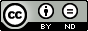### On the Question of Asymptotic Integration of Singularly Perturbed Fractional-Order Problems

Burkhan Kalimbetov

#### Abstract

In this paper we consider an initial problem for systems of differential equations of fractional order with a small parameter for the derivative. Regularization problem is produced, and algorithm for normal and unique solubility of general iterative systems of differential equations with partial derivatives is given.

#### Keywords

matrix-function, vector-function, differential equation of fractional order, regularization, asymptotic, iterative problems, normal and unique solvability.

PDF

#### References

Kalimbetov, B.T. and Safonov, V.F. (1995) A regularization method for systems with unstable spectral value of the kernel of the integral operator. Journal Differential equations, 31, 647-656.

Kalimbetov, B.T., Temirbekov, M.A. and Khabibullayev, Zh.O. (2012) Asymptotic solutions of singular perturbed problems with an instable spectrum of the limiting operator. Journal Abstract and Applied Analysis, 120192.

Katugampola, U. (2015) Correction to “What is a fractional derivative?” by Ortigueira and Machado. Journal Computational Physics, 293, 4–13.

R. Khalil, R., Al Horani, M., Yousef, A. and Sababheh, M. A new definition of fractional derivative. Journal Comput. Appl. Math., 264, 65–70.

Khalil, R., Anderson, D. and Al Horani, M. (2014) Undetermined coefficients for local fractional differential equations. URL: https://www.researchgate.net/publication/303903312.

Lomov, S.A. Introduction to General Theory of Singular Perturbations, 112, American Mathematical Society, Providence, USA. (1992)

DOI: https://doi.org/10.24203/ajfam.v6i3.5600

### Refbacks

• There are currently no refbacks.

Copyright (c) 2018 Asian Journal of Fuzzy and Applied Mathematics